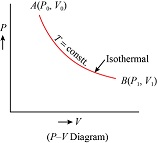Filter By

## All Questions

#### In a fluorescent lamp choke ( a small transformer) 100 V of reverse voltage is produced when the choke current changes uniformly from 0.25 A to 0 in a duration of 0.025 ms. The self-inductance of the choke (in mH) is estimated to be _____. Option: 1 10 Option: 2  20 Option: 3 30 Option: 4 40#### In an experiment for determination of refractive index of glass of a prism by, plot, it was found that a ray incident at angle 350, suffers a deviation of 400 and that it emerges at angle 790⋅  Ιn that case which of the following is closest to the maximum possible value of the refractive index ? Option: 1  1.5 Option: 2  1.6 Option: 3 1.7 Option: 4 1.8

This angle is greater than the 40° deviation angle already given, For greater μ, deviation will be even higher. Hence, μ of the given prism should be lesser than 1.5. Hence, the closest option will be 1.5.

## Crack CUET with india's "Best Teachers"

• HD Video Lectures
• Unlimited Mock Tests
• Faculty Support#### An ideal gas undergoes a quasi static, reversible process in which its molar heat capacity C remains constant.  If during  this process the relation of pressure P and volume V is given by PVn=constant,  then n is given by (Here CP and CV are molar specific heat at constant pressure and constant volume, respectively) Option: 1   Option: 2   Option: 3  Option: 4

For a polytropic preocess#### A point particle of mass m, moves along the uniformly rough track PQR as shown in the figure.  The coefficient of friction, between the particle and the rough track equals µ.  The particle is released, from rest, from the point P and it comes to rest at a point R.  The energies, lost by the ball, over the parts, PQ and QR, of the track, are equal to each other, and no energy is lost when particle changes direction from PQ to QR. The values of the coefficient of friction µ and the distance x(=QR), are, respectively close to :Option: 1  0.2 and 6.5 m   Option: 3  0.2 and 3.5 m   Option: 4   0.29 and 6.5 m

Work done by friction at QR = μmgx

In triangle, sin 30° = 1/2 = 2/PQ

PQ = 4 m

Work done by friction at PQ = μmg × cos 30° × 4 = μmg × √3/2 × 4 = 2√3μmg

Since work done by friction on parts PQ and QR are equal,

μmgx = 2√3μmg

x = 2√3 ≅ 3.5 m

Applying work energy theorem from P to R

decrease in P.E.=P.E.= loss of energy due to friction in PQPQ and QR

where h=2(given)

## Crack NEET with "AI Coach"

• HD Video Lectures
• Unlimited Mock Tests
• Faculty Support#### A particle of mass m is moving along the side of a square of side ‘a’, with a uniform speedin the x-y plane as shown in the figure :Which of the following statements is false for the angular momentumabout the origin? Option: 1 when the particle is moving from A to B. Option: 2 when the particle is moving from C to D. Option: 3 when the particle is moving from B to C. Option: 4when the particle is moving from D to A.

so option b is correct option

#### If a, b, c, d are inputs to a gate and x is its output, then, as per the following time graph, the gate is :Option: 1 NOT Option: 2 AND Option: 3 OR Option: 4 NAND \

The output of OR gate is 0 when all inputs are 0 and output is 1 when at least one of the input is 1.

Observing output x it is 0 when all inputs are 0 and it is 1 when at least one of the inputs is 1
therefore, the gate is OR

## Crack JEE Main with "AI Coach"

• HD Video Lectures
• Unlimited Mock Tests
• Faculty Support#### An ideal gas undergoes isothermal expansion at constant pressure.  During the process :Option: 1  enthalpy increases but entropy decreases.Option: 2  enthalpy remains constant but entropy increases.Option: 3  enthalpy decreases but entropy increases.Option: 4  Both enthalpy and entropy remain constant.#### A Laser light of wavelength 660 nm is used to weld Retina detachment.  If a Laser pulse of width 60 ms and power 0.5 kW is used the approximate number of photons in the pulse are : [Take Planck’s constant h=6.62×10−34 Js] Option: 1  1020 Option: 2 1018 Option: 3 1022 Option: 4 1019

As we discussed inor## Crack CUET with india's "Best Teachers"

• HD Video Lectures
• Unlimited Mock Tests
• Faculty Support#### For the P-V diagram given for an ideal gas,out of the following which one correctly represents the T-P diagram ?   Option: 1Option: 2Option: 3Option: 4PV=constant

The process is isothermal (temperature constant)

Also, from the process given, it can be seen that pressure is decreasing, P2<P1

Hence, the correct answer is Option (3)

#### A steel rail of length 5 m and area of cross section 40 cm2 is prevented from expanding along its length while the temperature rises by 100C.  If coefficient of linear expansion and Young’s modulus of steel are 1.2×10−5 K−1 and 2×1011 Nm−2 respectively, the force developed in the rail is approximately : Option: 1 2×107 N Option: 2  1×105 N Option: 3  2×109 N Option: 4  3×10−5 N